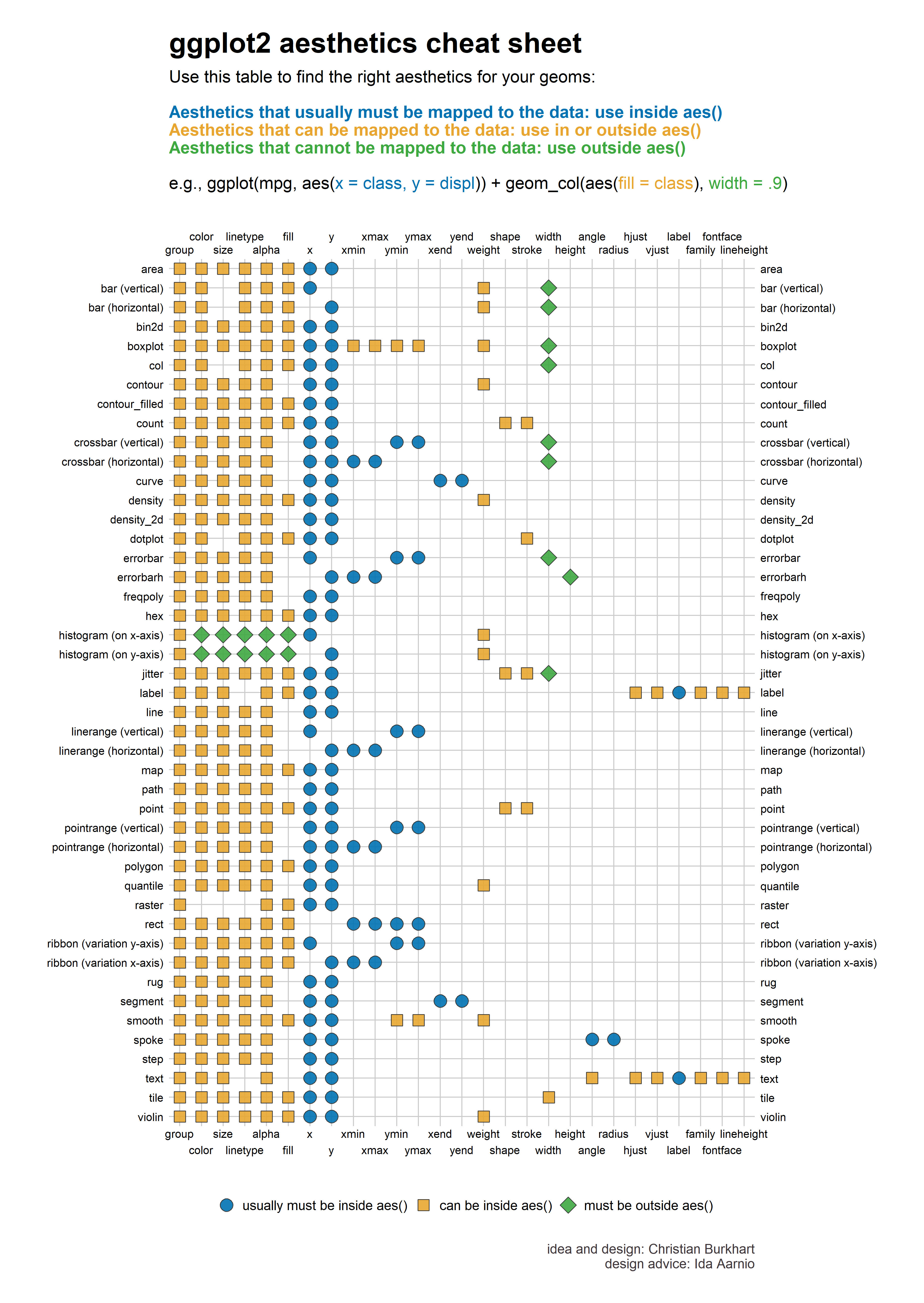# 第 27 章 ggplot2之统计图层

## 27.1 导言library(tidyverse)
library(palmerpenguins)

ggplot(data = penguins, mapping = aes(x = body_mass_g)) +
geom_histogram()• 映射到x轴的变量被分成了若干离散的小区间（bins)
• 需要计算每个小区间中有多少观测值落入其中
• 用于y轴上是一个新的变量
• 最终，用户提供的x变量和经过计算处理后的y变量，共同确定了柱状图中每个柱子的位置和高度

d <- tibble::tribble(
~variable, ~subject1, ~subject2, ~subject3,
"mass",         75,     70,    55,
"height",       154,    172,   144
)
d
## # A tibble: 2 × 4
##   variable subject1 subject2 subject3
##   <chr>       <dbl>    <dbl>    <dbl>
## 1 mass           75       70       55
## 2 height        154      172      144

geom_point(aes(x = mass, y = height)) 画图，却报错了。初学者可能苦苦搜索答案，然后被告知，ggplot画图需要先弄成tidy格式

d %>% pivot_longer(
cols = subject1:subject3,
names_to = "subject",
names_pattern = "subject(\\d)",
values_to = "value"
) %>%
pivot_wider(names_from = variable,
values_from = value)
## # A tibble: 3 × 3
##   subject  mass height
##   <chr>   <dbl>  <dbl>
## 1 1          75    154
## 2 2          70    172
## 3 3          55    144

## 27.2 为何及何时使用统计图层

“Even though the data is tidy, it may not represent the values you want to display”

simple_data <- tibble(group = factor(rep(c("A", "B"), each = 15)),
subject = 1:30,
score = c(rnorm(15, 40, 20), rnorm(15, 60, 10)))
simple_data
## # A tibble: 30 × 3
##   group subject score
##   <fct>   <int> <dbl>
## 1 A           1 43.8
## 2 A           2 50.7
## 3 A           3  6.29
## 4 A           4 55.7
## 5 A           5 73.6
## 6 A           6 50.2
## # … with 24 more rows

simple_data %>%
group_by(group) %>%
summarize(
mean_score = mean(score),
.groups = 'drop'
) %>%
ggplot(aes(x = group, y = mean_score)) +
geom_col()simple_data %>%
group_by(group) %>%
summarize(
mean_score = mean(score),
.groups = 'drop'
) 
## # A tibble: 2 × 2
##   group mean_score
##   <fct>      <dbl>
## 1 A           46.4
## 2 B           62.3

simple_data %>%
group_by(group) %>%
summarize(
mean_score = mean(score),
se = sqrt(var(score)/length(score)),
.groups = 'drop'
) %>%
mutate(
lower = mean_score - se,
upper = mean_score + se
)
## # A tibble: 2 × 5
##   group mean_score    se lower upper
##   <fct>      <dbl> <dbl> <dbl> <dbl>
## 1 A           46.4  5.33  41.1  51.8
## 2 B           62.3  2.58  59.7  64.9

simple_data %>%
group_by(group) %>%
summarize(
mean_score = mean(score),
se = sqrt(var(score)/length(score)),
.groups = 'drop'
) %>%
mutate(
lower = mean_score - se,
upper = mean_score + se
) %>%
ggplot(aes(x = group, y = mean_score, ymin = lower, ymax = upper)) +
geom_errorbar()simple_data_bar <- simple_data %>%
group_by(group) %>%
summarize(
mean_score = mean(score),
.groups = 'drop'
)

simple_data_errorbar <- simple_data %>%
group_by(group) %>%
summarize(
mean_score = mean(score),
se = sqrt(var(score)/length(score)),
.groups = 'drop'
) %>%
mutate(
lower = mean_score - se,
upper = mean_score + se
)

ggplot() +
geom_col(
aes(x = group, y = mean_score),
data = simple_data_bar
) +
geom_errorbar(
aes(x = group, y = mean_score, ymin = lower, ymax = upper),
data = simple_data_errorbar
)OMG, 为了画一个简单的图，我们需要写这么长的一段代码。究其原因就是，我们认为，一定要准备好一个tidy的数据，并且把想画的几何形状所需要的美学映射，都整理到这个tidy的数据框中

simple_data %>%
ggplot(aes(group, score)) +
stat_summary(geom = "bar") +
stat_summary(geom = "errorbar")Bingo

## 27.3 用 stat_summary() 理解统计图层

height_df <- tibble(group = "A",
height = rnorm(30, 170, 10))

height_df %>%
ggplot(aes(x = group, y = height)) +
geom_point()height_df %>%
ggplot(aes(x = group, y = height)) +
stat_summary()• x or y
• ymin or xmin
• ymax or xmax

No summary function supplied, defaulting to mean_se()

• 首先，对于stat_summary()中的fun.data参数，它的默认值是mean_se()
• 其次，我们看看这个函数
mean_se
function (x, mult = 1)
{
x <- stats::na.omit(x)
se <- mult * sqrt(stats::var(x)/length(x))
mean <- mean(x)
new_data_frame(list(y = mean, ymin = mean - se, ymax = mean +
se), n = 1)
}
<bytecode: 0x0000021aef28aa10>
<environment: namespace:ggplot2>

• 删除缺失值NA
• 计算出se, 公式为$$SE = \sqrt{\frac{1}{N}\sum_{i=1}^N(x_i-\bar{x})^2}$$
• 计算x的均值
• 创建一个数据框（一行三列），y = mean, ymin = mean - se, ymax = mean + se

##     y  ymin  ymax
## 1 170 168.2 171.7

pointrange_plot <- height_df %>%
ggplot(aes(x = group, y = height)) +
stat_summary()

layer_data(pointrange_plot, 1)
##   x group   y  ymin  ymax PANEL flipped_aes colour
## 1 1     1 170 168.2 171.7     1       FALSE  black
##   size linetype shape fill alpha stroke
## 1  0.5        1    19   NA    NA      1

### 27.3.1 小结

• 函数stat_summary()里若没有指定数据，那就会从ggplot(data = .)里继承
• 参数fun.data 会调用函数将数据变形，这个函数默认是mean_se()
• fun.data 返回的是数据框，这个数据框将用于geom参数画图，这里缺省的geom是pointrange
• 如果fun.data 返回的数据框包含了所需要的美学映射，图形就会显示出来。

height_df %>%
ggplot(aes(x = group, y = height)) +
stat_summary(
geom = "pointrange",
fun.data = mean_se
)Look, it’s the same plot!

## 27.4 使用统计图层

### 27.4.1 包含95%置信区间的误差棒

my_penguins <- na.omit(penguins)

my_penguins %>%
ggplot(aes(sex, body_mass_g)) +
stat_summary(
fun.data = ~mean_se(., mult = 1.96), # Increase mult value for bigger interval!
geom = "errorbar",
)female_mean_se <- my_penguins %>%
filter(sex == "female") %>%
pull(body_mass_g) %>%
mean_se(., mult = 1.96)

male_mean_se <- my_penguins %>%
filter(sex == "male") %>%
pull(body_mass_g) %>%
mean_se(., mult = 1.96)

bind_rows(female_mean_se, male_mean_se)
##      y ymin ymax
## 1 3862 3761 3964
## 2 4546 4427 4665

ggplot()中提供了分组变量（比如这里的sex），stat_summary()会分组计算， 再次感受到ggplot2的强大气息！

### 27.4.2 带有彩色填充色的柱状图

calc_median_and_color <- function(x, threshold = 40) {
tibble(y = median(x)) %>%
mutate(fill = ifelse(y < threshold, "pink", "grey35"))
}

my_penguins %>%
ggplot(aes(species, bill_length_mm)) +
stat_summary(
fun.data = calc_median_and_color,
geom = "bar"
)my_penguins %>%
group_split(species) %>%
map(~ pull(., bill_length_mm)) %>%
map_dfr(calc_median_and_color)
## # A tibble: 3 × 2
##       y fill
##   <dbl> <chr>
## 1  38.8 pink
## 2  49.6 grey35
## 3  47.4 grey35

### 27.4.3 大小变化的点线图

my_penguins %>%
ggplot(aes(species, bill_depth_mm)) +
stat_summary(
fun.data = function(x) {

scaled_size <- length(x)/nrow(my_penguins)

mean_se(x) %>%
mutate(size = scaled_size)
}
)my_penguins %>%
group_split(species) %>%
map(~ pull(., bill_depth_mm)) %>%
map_dfr(
function(x) {

scaled_size <- length(x)/nrow(my_penguins)

mean_se(x) %>%
mutate(size = scaled_size)
}
)
##       y  ymin  ymax   size
## 1 18.35 18.25 18.45 0.4384
## 2 18.42 18.28 18.56 0.2042
## 3 15.00 14.91 15.09 0.3574

## 27.5 总结

### 27.5.1 主要结论

• 尽管数据是tidy的，但它未必能代表你想展示的值

• 解决办法不是去规整数据以符合几何形状的要求，而是将原初tidy数据传递给ggplot(), 让stat_*()函数在内部实现变型

• 可以stat_*()函数可以定制geom以及相应的变形函数。当然，定制自己的函数，需要核对stat_*()所需要的变量和数据类型

• 如果想用不同的geom，确保变换函数能计算出(几何形状所需要的)美学映射

### 27.5.2 STAT vs. GEOM or STAT and GEOM?

layer()分成stat_*()geom_*()两块，或许是一个失误，最后我们用Hadley的原话来结束本章内容

Unfortunately, due to an early design mistake I called these either stat_() or geom_(). A better decision would have been to call them layer_() functions: that’s a more accurate description because every layer involves a stat and a geom